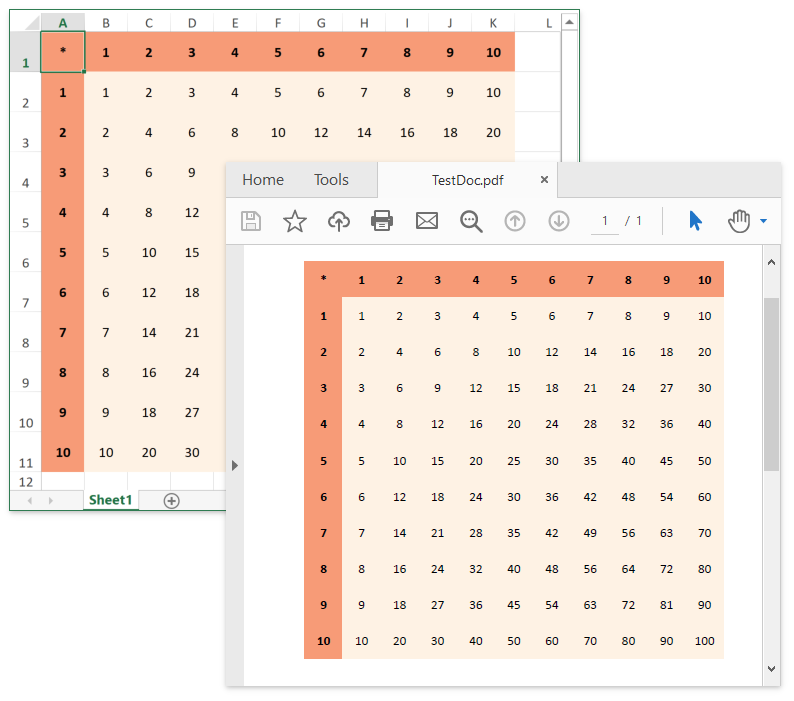The requested page is not available for the requested platform. You are viewing the content for .NET Framework 4.5.2+ platform.

Mobile

19.2 (EAP/Beta)
19.1
18.2
18.1
17.2
.NET Framework 4.5.2+
.NET Framework 4.5.2+
.NET Standard 2.0+

Getting Started

• 8 min to read
Important

You require a license to the DevExpress Office File API or DevExpress Universal Subscription to use these examples in production code. Refer to the DevExpress Subscription page for pricing information.

Perform the following steps to get started with the non-visual spreadsheet component:

1. Start Visual Studio and create a new Console Application (NET Framework) project.

2. Add references to the following libraries:

• DevExpress.Charts.v19.1.Core.dll
• DevExpress.Data.v19.1.dll
• DevExpress.DataAccess.v19.1.dll
• DevExpress.Docs.v19.1.dll
• DevExpress.Office.v19.1.Core.dll
• DevExpress.Pdf.v19.1.Core.dll
• DevExpress.Printing.v19.1.Core.dll
• DevExpress.Sparkline.v19.1.Core.dll
• DevExpress.XtraCharts.v19.1.dll
3. Paste the code below in the Main method of the Program.cs file (Main procedure of the Module1.vb file for Visual Basic).

// ...

// Create an instance of a workbook.
Workbook workbook = new DevExpress.Spreadsheet.Workbook();

// Access the first worksheet in the workbook.
Worksheet worksheet = workbook.Worksheets;

// Access the "A1" cell in the worksheet.
Cell cell = worksheet.Cells["A1"];

// Specify the "A1" cell value.
cell.Value = 1;

// Fill cells with sequential numbers by using shared formulas.
worksheet.Range["A2:A10"].Formula = "=SUM(A1+1)";
worksheet.Range["B1:B10"].Formula = "=A1+2";

// Multiply values contained in the cell range A1 through A10
// by the corresponding values contained in B1 through B10,
// and display the results in cells C1 through C10.
worksheet.Range["C1:C10"].ArrayFormula = "=A1:A10*B1:B10";

// Save the document file under the specified name.
workbook.SaveDocument("TestDoc.xlsx", DocumentFormat.OpenXml);

// Display gridlines in PDF.
worksheet.PrintOptions.PrintGridlines = true;

// Export the document to PDF.
workbook.ExportToPdf("TestDoc.pdf");

// Open the PDF document using the default viewer.
System.Diagnostics.Process.Start("TestDoc.pdf");

// Open the XLSX document using the default application.
System.Diagnostics.Process.Start("TestDoc.xlsx");

// Create an instance of a workbook.
Workbook workbook = new DevExpress.Spreadsheet.Workbook();

// Access the first worksheet in the workbook.
Worksheet worksheet = workbook.Worksheets;

// Access the "A1" cell in the worksheet.
Cell cell = worksheet.Cells["A1"];

// Specify the "A1" cell value.
cell.Value = 1;

// Fill cells with sequential numbers by using shared formulas.
worksheet.Range["A2:A10"].Formula = "=SUM(A1+1)";
worksheet.Range["B1:B10"].Formula = "=A1+2";

// Multiply values contained in the cell range A1 through A10
// by the corresponding values contained in B1 through B10,
// and display the results in cells C1 through C10.
worksheet.Range["C1:C10"].ArrayFormula = "=A1:A10*B1:B10";

// Save the document file under the specified name.
workbook.SaveDocument("TestDoc.xlsx", DocumentFormat.OpenXml);

// Display gridlines in PDF.
worksheet.PrintOptions.PrintGridlines = true;

// Export the document to PDF.
workbook.ExportToPdf("TestDoc.pdf");

// Open the PDF document using the default viewer.
var pdfProcess = new Process();
pdfProcess.StartInfo = new ProcessStartInfo("TestDoc.pdf");
pdfProcess.StartInfo.UseShellExecute = true;
pdfProcess.Start();

// Open the XLSX document using the default application.
Process.Start(new ProcessStartInfo("TestDoc.xlsx"){UseShellExecute = true});

4. Run the project.

The following image displays files generated after the above-mentioned code is executed.1. Start Visual Studio and create a new Console Application (NET Core) project.

2. Install the DevExpress.Document.Processor NuGet package.

3. Paste the code below in the Main method of the Program.cs file (Main procedure of the Module1.vb file for Visual Basic).

// ...

// Create an instance of a workbook.
Workbook workbook = new DevExpress.Spreadsheet.Workbook();

// Access the first worksheet in the workbook.
Worksheet worksheet = workbook.Worksheets;

// Access the "A1" cell in the worksheet.
Cell cell = worksheet.Cells["A1"];

// Specify the "A1" cell value.
cell.Value = 1;

// Fill cells with sequential numbers by using shared formulas.
worksheet.Range["A2:A10"].Formula = "=SUM(A1+1)";
worksheet.Range["B1:B10"].Formula = "=A1+2";

// Multiply values contained in the cell range A1 through A10
// by the corresponding values contained in B1 through B10,
// and display the results in cells C1 through C10.
worksheet.Range["C1:C10"].ArrayFormula = "=A1:A10*B1:B10";

// Save the document file under the specified name.
workbook.SaveDocument("TestDoc.xlsx", DocumentFormat.OpenXml);

// Display gridlines in PDF.
worksheet.PrintOptions.PrintGridlines = true;

// Export the document to PDF.
workbook.ExportToPdf("TestDoc.pdf");

// Open the PDF document using the default viewer.
System.Diagnostics.Process.Start("TestDoc.pdf");

// Open the XLSX document using the default application.
System.Diagnostics.Process.Start("TestDoc.xlsx");

// Create an instance of a workbook.
Workbook workbook = new DevExpress.Spreadsheet.Workbook();

// Access the first worksheet in the workbook.
Worksheet worksheet = workbook.Worksheets;

// Access the "A1" cell in the worksheet.
Cell cell = worksheet.Cells["A1"];

// Specify the "A1" cell value.
cell.Value = 1;

// Fill cells with sequential numbers by using shared formulas.
worksheet.Range["A2:A10"].Formula = "=SUM(A1+1)";
worksheet.Range["B1:B10"].Formula = "=A1+2";

// Multiply values contained in the cell range A1 through A10
// by the corresponding values contained in B1 through B10,
// and display the results in cells C1 through C10.
worksheet.Range["C1:C10"].ArrayFormula = "=A1:A10*B1:B10";

// Save the document file under the specified name.
workbook.SaveDocument("TestDoc.xlsx", DocumentFormat.OpenXml);

// Display gridlines in PDF.
worksheet.PrintOptions.PrintGridlines = true;

// Export the document to PDF.
workbook.ExportToPdf("TestDoc.pdf");

// Open the PDF document using the default viewer.
var pdfProcess = new Process();
pdfProcess.StartInfo = new ProcessStartInfo("TestDoc.pdf");
pdfProcess.StartInfo.UseShellExecute = true;
pdfProcess.Start();

// Open the XLSX document using the default application.
Process.Start(new ProcessStartInfo("TestDoc.xlsx"){UseShellExecute = true});

4. Run the project.

The following image displays files generated after the above-mentioned code is executed.